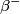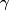## Solution to 1986 Problem 17

 (A) This is the correct answer. Note that the antineutrino is needed to conserve electron number in the first stage.(B) Because of conservation of electron number,$\beta^-$ emission cannot occur without emission of a neutrino as well. For the same reason$\alpha$ emission with a neutrino would violate conservation of lepton number.(C) Because of conservation of electron number,$\beta^-$ emission cannot occur without emission of a neutrino as well. Also, a$\gamma$ is emitted when a nucleus in an excited state drops down to a less-excited state. This process does not change the number of nucleons or the number of protons. So, neither of these processes could change the number of nucleons in the nucleus.(D) Deuteron emission is not a form of natural radioactivity.(E) A$\gamma$ is emitted when a nucleus in an excited state drops down to a less-excited state. This process does not change the number of nucleons or the number of protons.